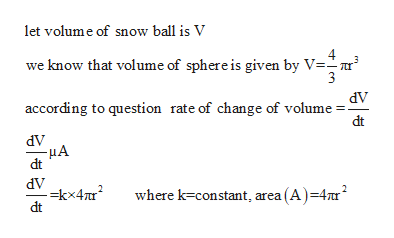# A spherical snowball melts at a rate proportional to its surface area. Show that the rate of change of the radius is constant. (hint: surface area: 4(pi)r^2)

Question
127 views

A spherical snowball melts at a rate proportional to its surface area. Show that the rate of change of the radius is constant. (hint: surface area: 4(pi)r^2)

check_circle

Step 1

Given that spherical snow ball melts at a rate proportional to its surface area

Show that rate of change of radius is constanthelp_outlineImage Transcriptioncloselet volume of snow ball is V 4 know that volume of sphere is given by V=r 3 dV according to question rate of change of volume dt dV dV -=kx4 dt where k constant, area (A ) =4ur2 fullscreen
Step 2

It is kwon that volume is a function of r...

### Want to see the full answer?

See Solution

#### Want to see this answer and more?

Solutions are written by subject experts who are available 24/7. Questions are typically answered within 1 hour.*

See Solution
*Response times may vary by subject and question.
Tagged in